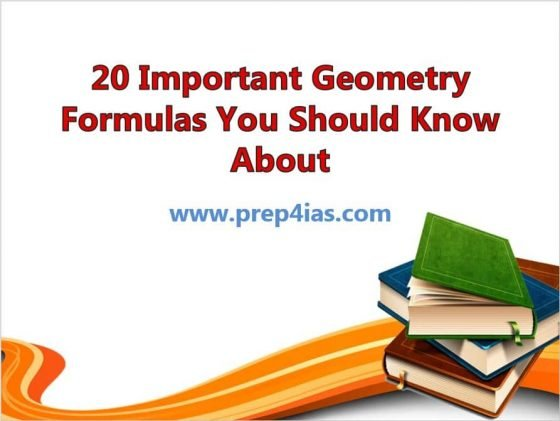# 20 Important Geometry Formulas You Should Know About

In this article, we will look into 20 Important Geometry Formulas You Should Know About. In a mathematical world, there are few basic geometry formulas which are important for everyone to understand and remember so that it can be used to solve the complex problems in multiple streams. Whether it is for academic purposes or for solving other technical problems, one can find the application of these formulas almost everywhere in their life. More on wikipedia.## 20 Important Geometry Formulas You Should Know About

### 1. How to find the area of a Circle ?

Ans. The area of a circle is given as,where, r = radius of circle.

### 2. How to find the circumference of a Circle ?

Ans. The circumference of a circle is given as,where, r = radius of the circle.

### 3. How to find the area of a rectangle ?

Ans. The area of a rectangle is given as,w = width of the rectangle

l = length of the rectangle

### 4. How to find the perimeter of a rectangle ?

Ans. The perimeter of a rectangle is given as,l = length of the rectangle

w = width of the rectangle.

### 5. How to find the perimeter of a square ?

Ans. The perimeter of a square is given as,where, a = side of the square.

### 6. How to find the area of a square ?

Ans. The area of a square is given as,where, a = side of the square.

### 7. How to find the volume of a sphere ?

Ans. The volume of a sphere is given as,where, r = radius of the sphere.

### 8. How to find the surface area of a sphere ?

Ans. The surface area of a sphere is given as,where, r = radius of the sphere.

### 9. How to find the area of a triangle ?

Ans. The area of a triangle is given as,h = height of the triangle

b = base of the triangle

### 10. How to find the perimeter of a triangle ?

Ans. The perimeter of a triangle is given as,a = side of the triangle

b = base of the triangle

c = side of the triangle

### 11. How to find the volume of a cube ?

Ans. The volume of a cube is given as,where, a = edge of the cube

### 12. How to find the surface area of a cube ?

Ans. The surface area of a cube is given as,where, a = edge of the cube

### 13. How to find the surface area of a right circular cone ?

Ans. The surface area of a right circular cone is given as,h = height

### 14. How to find the area of an equilateral triangle ?

Ans. The area of an equilateral triangle is given as,where, a = side of the equilateral triangle

### 15. How to find the area of an isosceles triangle ?

Ans. The area of an isosceles triangle is given as,b = base of the triangle

h = height of the triangle

### 16. How to find the area of a parallelogram ?

Ans. The area of a parallelogram is given as,b = base of the parallelogram

h = height of the parallelogram

### 17. How to find the area of a rhombus ?

Ans. The area of a rhombus is given as,p = length of diagonal 1

q = length of diagonal 2

### 18. How to find the volume of a cylinder ?

Ans. The volume of a cylinder is given as,r = radius of the cylinder

h = height of the cylinder

### 19. How to find the surface area of a cylinder ?

Ans. The surface area of a cylinder is given as,r = radius of the cylinder

h = height of the cylinder

### 20. How to find the area of a trapezium ?

Ans. The area of a trapezium is given as,a,b = bases of trapezium

h = height(perpendicular distance between a and b)